# Pythagoras Theorem

Pythagoras Theorem is an important topic in Maths, which explains the relation between the sides of a right-angled triangle. It is also sometimes called the Pythagorean Theorem. The formula and proof of this theorem are explained here with examples. This theorem is basically used for the right-angled triangle and by which we can derive base, perpendicular and hypotenuse formula. Let us learn this theorem in detail here.

## Pythagoras Theorem Statement

Pythagoras theorem states that “In a right-angled triangle,  the square of the hypotenuse side is equal to the sum of squares of the other two sides“. The sides of this triangle have been named as Perpendicular, Base and Hypotenuse. Here, the hypotenuse is the longest side, as it is opposite to the angle 90°. The sides of a right triangle (say a, b and c) which have positive integer values, when squared, are put into an equation, also called a Pythagorean triple.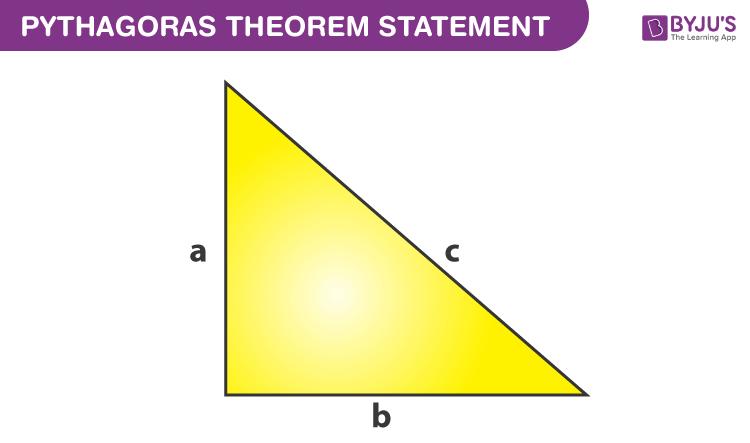### History

The theorem is named after a greek Mathematician called Pythagoras.

## Pythagoras Theorem Formula

Consider the triangle given above:

Where “a” is the perpendicular side,

“b” is the base,

“c” is the hypotenuse side.

According to the definition, the Pythagoras Theorem formula is given as:

 Hypotenuse2 = Perpendicular2 + Base2  c2 = a2 + b2

The side opposite to the right angle (90°)  is the longest side (known as Hypotenuse) because the side opposite to the greatest angle is the longest.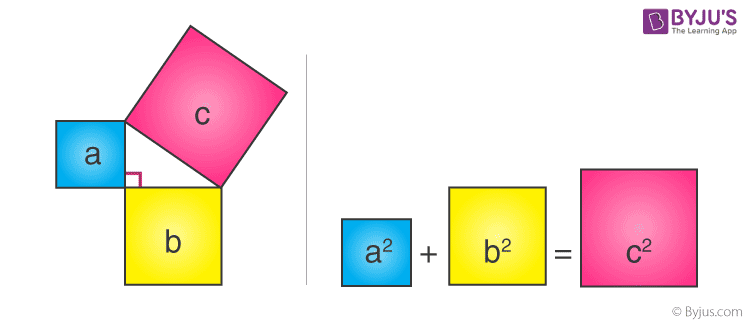Consider three squares of sides a, b, c mounted on the three sides of a triangle having the same sides as shown.

By Pythagoras Theorem –

Area of square A + Area of square B = Area of square C

### Example

The examples of theorem based on the statement given for right triangles is given below:

Consider a right triangle, given below: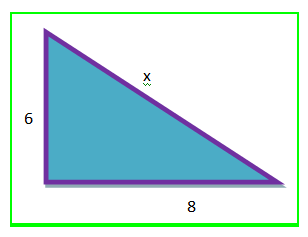Find the value of x.

X is the side opposite to right angle, hence it is a hypotenuse.

Now, by the theorem we know;

Hypotenuse2 = Base2 + Perpendicular2

x2 = 82 + 62

x2 = 64+36 = 100

x = √100 = 10

Therefore, we found the value of hypotenuse here.

## Pythagoras Theorem Proof

Given: A right-angled triangle ABC, right-angled at B.

To Prove- AC2 = AB2 + BC2

Construction: Draw a perpendicular BD meeting AC at D.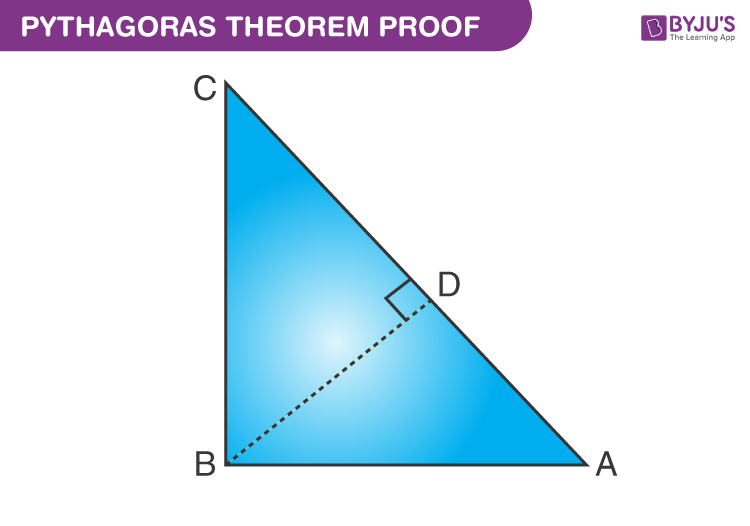Proof:

Therefore, $\frac{AD}{AB}=\frac{AB}{AC}$ (corresponding sides of similar triangles)

Or, AB= AD × AC ……………………………..……..(1)

Also, △BDC ~△ABC

Therefore, $\frac{CD}{BC}=\frac{BC}{AC}$ (corresponding sides of similar triangles)

Or, BC2= CD × AC ……………………………………..(2)

Adding the equations (1) and (2) we get,

AB+ BC= AD × AC + CD × AC

AB+ BC= AC (AD + CD)

Since, AD + CD = AC

Therefore, AC2 = AB2 + BC2

Hence, the Pythagorean theorem is proved.

Note: Pythagorean theorem is only applicable to Right-Angled triangle.## Applications of Pythagoras Theorem

• To know if the triangle is a right-angled triangle or not.
• In a right-angled triangle, we can calculate the length of any side if the other two sides are given.
• To find the diagonal of a square.

### Useful For

Pythagoras theorem is useful to find the sides of a right-angled triangle. If we know the two sides of a right triangle, then we can find the third side.

### How to use?

To use this theorem, remember the formula given below:

c2 = a2 + b2

Where a, b and c are the sides of the right triangle.

For example, if the value of a = 3 cm, b = 4 cm, then find the value of c.

We know,

c2 = a2 + b2

c2 = 32+42

c= 9+16

c= 25

c = √25

c = 5

Hence, the third side is 5 cm.

As we can see, a + b > c

3 + 4 > 5

7 > 5

Hence, c = 5 cm is the hypotenuse of the given triangle.

### How to find whether a triangle is a right-angled triangle?

If we are provided with the length of three sides of a triangle, then to find whether the triangle is a right-angled triangle or not, we need to use the Pythagorean theorem.

Let us understand this statement with the help of an example.

Suppose a triangle with sides 10, 24, and 26 are given.

Clearly, 26 is the longest side.

It also satisfies the condition, 10 + 24 > 26

We know,

c= a+ b2    ………(1)

So, let a = 10,b = 24 and c = 26

First we will solve R.H.S. of equation 1.

a2 + b2 = 102 + 242 = 100 + 576 = 676

Now, taking L.H.S, we get;

c2 = 262 = 676

We can see,

LHS = RHS

Therefore, the given triangle is a right triangle, as it satisfies the theorem.

## Pythagorean Theorem Problems

Problem 1: The sides of a triangle are 5,12 & 13 units. Check if it has a right angle or not.

Solution: From Pythagoras Theorem, we have;

Perpendicular2 + Base2 = Hypotenuse2

Let,

Perpendicular = 12 units

Base = 5 units

Hypotenuse = 13 units

122 + 52 = 132

⇒ 144 + 25 = 169

⇒ 169 = 169

L.H.S. = R.H.S.

Therefore, the angle opposite to the 13 unit side will be at a right angle.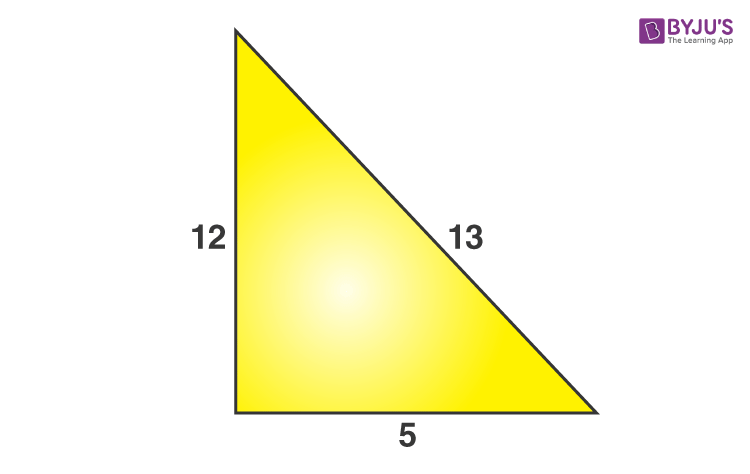Problem 2: The two sides of a right-angled triangle are given as shown in the figure. Find the third side.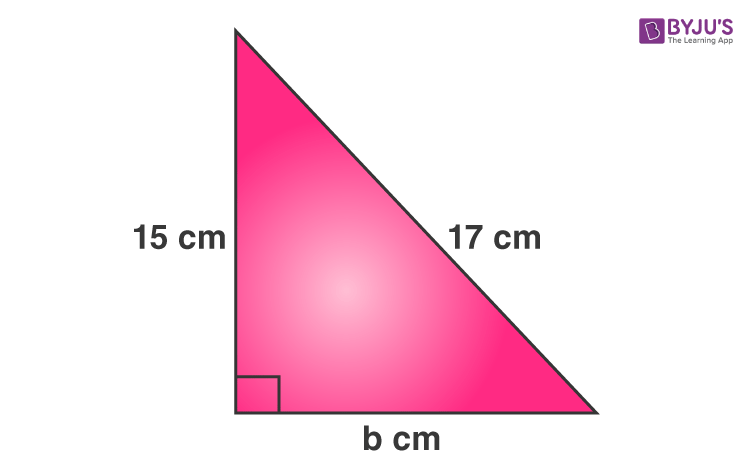Solution: Given;

Perpendicular = 15 cm

Base = b cm

Hypotenuse = 17 cm

As per the Pythagorean Theorem, we have;

Perpendicular2 + Base2 = Hypotenuse2

⇒152 + b2 = 172

⇒225 + b2 = 289

⇒b2 = 289 – 225

⇒b2 = 64

⇒b = √64

Therefore, b = 8 cm

Problem 3: Given the side of a square to be 4 cm. Find the length of the diagonal.

Solution- Given;

Sides of a square = 4 cm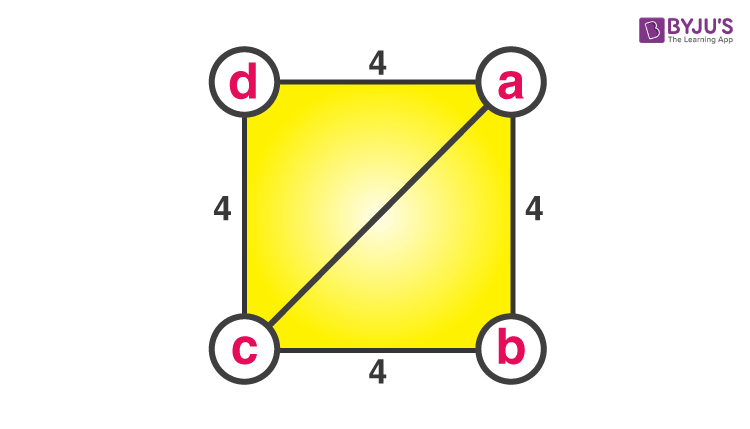To Find- The length of diagonal ac.

Consider triangle abc (or can also be acd)

(ab)2 +(bc)= (ac)2

(4)2 +(4)2= (ac)2

16 + 16 = (ac)2

32 = (ac)2

(ac)2 = 32

ac = 4√2.

Thus, the length of the diagonal is 4√2 cm.

Stay tuned with BYJU’S – The Learning App to learn all the important mathematical concepts and also watch interactive videos to learn with ease.

## Frequently Asked Questions – FAQs

### What is the formula for Pythagorean Theorem?

The formula for Pythagoras, for a right-angled triangle, is given by; c2=a2+b2

### What is the formula for hypotenuse?

The hypotenuse is the longest side of the right-angled triangle, opposite to right angle, which is adjacent to base and perpendicular. Let base, perpendicular and hypotenuse be a, b and c respectively. Then the hypotenuse formula, from the Pythagoras statement will be;
c = √(a2 + b2)

### Can we apply the Pythagoras Theorem for any triangle?

No, this theorem is applicable only for the right-angled triangle.

### What is an example of Pythagoras theorem?

An example of using this theorem is to find the length of the hypotenuse given the length of the base and perpendicular of a right triangle.

### What is the use of Pythagoras theorem?

The theorem can be used to find the steepness of the hills or mountains. To find the distance between the observer and a point on the ground from the tower or a building above which the observer is viewing the point. It is mostly used in the field of construction.

1. Rohit

I want all before year question papers of 10th cbse please send me as soon as possible my exams are going to be start

1. lavanya
2. Erika

Hey at least you could have said please
And they are not just any company a very successful and good and busy one
So I don’t they will even see your question and write back(I am sure)
I suggest you go to Byju’s query and type in your question .you will get your answers as soon as possible (I am telling this to you even though that website is just for Byjuians,the people who has taken the Byjus subscription)
And you can also take the byjus subscription

2. Joshua

I could understand this concept very well even though I’m in sixth grade. I learnt this for my math project.
It was very helpful. thanks to Byju’ s.

3. Arya

Please explain about pythogorean theorem for side in detail for the project

1. lavanya
4. Sahib Jeet Singh

I think that we children can use this website very well and it is also very helpful for us and I have used this website for the first time By the way I liked everything.

1. Sweet das

Yes I am satisfied this link 😊.

yes l am satisfied this link

6. Erika

U know I have Byju’s subscription by the way
And the explanations are just too good
I get near full marks now for this
And the people who are requesting the questions you will not get answers as they are a very busy company
They are just not any company you know very (very very very very very very very)successful ones

7. ayo

Thanks to this website I will be the best student in my class thanks BYJUS I really appreciate it

8. Thanmayi

Thank you very much byju’s for this. It really helped me in my math project.

9. Ana Angela

Useful page and helped me understanding the concepts formulas I hope for much betterment